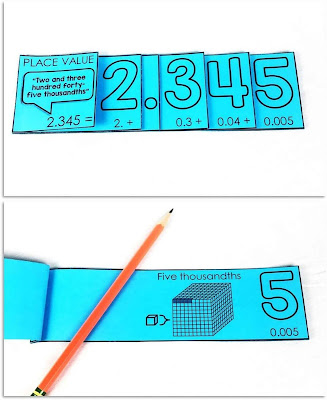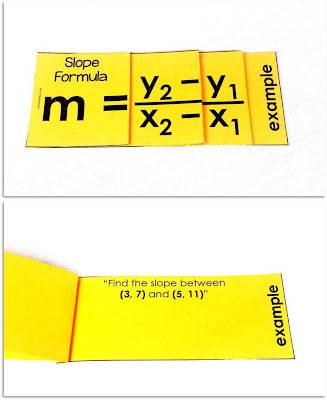## Pages

### 7 Math Interactive Notebook Foldables

"Miss, why are we cutting and pasting? Do you think we're babies?"

Then within a few weeks, my consumer math seniors would be pretty into building their interactive notebooks. And not one student lost a notebook over the years. I believe this ownership was built along with the cutting, coloring, arranging and pasting. Their notebooks became something my students didn't want to lose because of all the work they represented. Though the cutting and pasting does take more time than typical math notes, I feel this tradeoff is well worth it.Linear equations foldables

Do your students enjoy building interactive notebooks? Or maybe you haven't yet tried them and are interested in learning about the benefits. To me, that ownership is the main benefit. Kids like and deserve getting creative, and math is a good a place as any for a little creativity.

In this post, I want to show you some of the interactive notebook foldables I've made over the years for students of all ages. They can all stand alone as flipbooks. Each comes together with scissors and a stapler.

### MATH FOLDABLES

This place value foldable highlights what exactly the expanded form of a number means.Place value foldable

Under each flap is a visual representation of the place value (above you can see 5 blocks of 1000 to represent 5000) and a piece of the number's expanded form.

Decimals are so weird to visualize. Underneath each flap of this decimal place value foldable is a visual representation of how small these tenths, hundredths, thousandths get in relation to a unit cube.Decimal place value foldable

A thousandth is really small! It really becomes concrete seeing how small 0.005 is compared to a unit cube.

This slope foldable comes in a larger set of linear equations foldables that includes the slope formula, the point-slope form of a linear equation and the slope-intercept form of a linear equation.Slope foldable

In this photo you can see the scaled-down version of the foldable that students can use to take notes. There is also a version that is more scaffolded along with a digital version in Google Slides. The printable foldables can be glued into student notebooks or can stand alone as references for the equations.

This one takes more than scissors and a staple to go together, but if your students are like most and struggle with slope, this comparing slope foldable lets them see and feel it.

Our quadratics unit was always one of my favorites to teach. Kids would start our unit totally intimidated but end as quadratic bosses. I loved being there for that growth.Quadratic word problems foldable

This quadratic word problems foldable helps students identify the important parts of a parabola as they work through projectile motion problems.

This quadratics in vertex form foldable comes in a larger set of nonlinear functions in vertex form foldables. Included in the set is this one for quadratics, along with one for absolute value functions in vertex form and radical functions in vertex form.Quadratic functions foldable

Under each flap is an explanation of the a value, that x stays as x, which way h shifts, to remember to square the parenthesis, and which way k shifts.

I loved teaching consumer math because of how learning to be smart with money was so empowering. This compound interest foldable is part of a larger set that includes a simple interest foldable, an annual compound interest foldable and this one where interest is compounded multiple times throughout the year.Compound interest foldable

I put parenthesis around the exponent to remind students that n and t are multiplied first. This was the #1 issue I'd see when students worked with this formula.

You can see all of the foldables I've made here: Math FoldablesFree math resource library